# Understanding pips and pipettes in forex trading

###### Desmond LeongIf you are new to the world of forex trading, you may be wondering what a pip is. Short for "points in percentage", pips are the smallest incremental move that a currency pair can make.

'Pips', 'spreads', and 'pipettes', are all common forex terms that new aspiring forex traders need to wrap their heads around.

In this guide, we will explain how a pip works, how to calculate a pip and what's the difference between a pip and a pipette.

Keep reading and take your time with this information, it is critical knowledge for all new traders entering the market to know exactly how forex trading works.

## What is a pip in forex?

A pip is the standardised unit measuring a change (both gains and losses) of a currency pair in the forex market. It is the smallest increment in the value of an exchange rate between a currency pair.

A pip, also known as a "point" in currency trading, is worth 1/100th of one cent on most exchanges. Forex traders typically use pips to calculate profits and losses when dealing with forex trading transactions.

### What does pip stand for?

'Pip' can stand for 'percentage in point' or 'price interest point' within the forex market.

## How do pips work?

A pip measures the amount of change in the exchange rate of a currency pair, calculated using its 4th decimal (in JPY pairs, it is calculated using the 2nd decimal).

It is important to note that pips do not represent any actual cash value - that depends on the position size of the trade, which would affect the pip value.

#### Example of a pip using a major currency pair

In the EUR/USD currency pair, pip movement from 1.1080 to 1.1081 is an increase of 1 pip.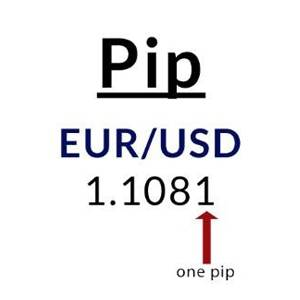Pip is calculated using the 4th decimal point

A trader that is going to buy the EUR/USD will profit if the euro increases in value against the US dollar. If the trade was entered at 1.1081 and exited at 1.1126, they would have made:

1.1126 - 1.1081 = 45 pips

However, if the trade went the opposite way, the trader would have suffered a loss.

#### Example of a pip using Japanese Yen pairs

In the USD/JPY currency pair, pip movement from 10.44 to 10.43 is a decrease of 1 pip.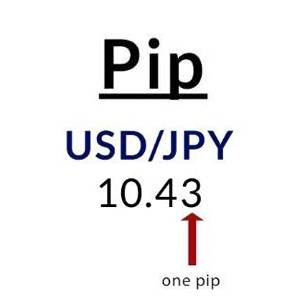Pip is calculated using the 2nd decimal point in Japanese Yen pairs

A trader that is going to buy the USD/JPY will profit if the dollar increases in value against the Japanese Yen. If the trade was entered at 10.43 and exited at 10.96, they would have made:

10.96 - 10.43 = 53 pips

If the market went the opposite way then the trader would have seen a loss.

## What is a pipette in forex?

Pipettes are fractional pips. It is 1/10 of a pip, usually calculated using the 5th decimal (in JPY pairs, it is calculated using the 3rd decimal).

#### Example of a pipette using a major currency pair

In the EUR/USD currency pair, a movement from 1.10811 to 1.10812 is an increase of 1 pipette.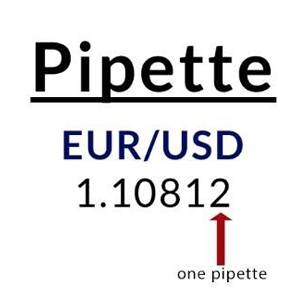Pipette is calculated using the 5th decimal point

#### Example of a pipette using a Japanese Yen currency pair

In the USD/JPY currency pair, a movement from 10.433 to 10.432 is a decrease of 1 pipette.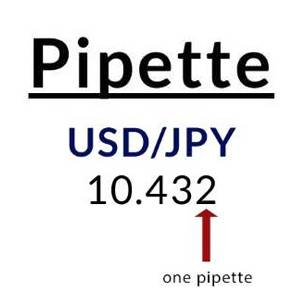Pipette is calculated using the 3rd decimal point in Japanese Yen pairs

## What is the pip value?

A pip value is defined by the currency pair being traded, the exchange rate of the pair, and the size of the trade.

The pip value is usually referred to when referencing the performance of a position to attribute price to forex trade, whether it's a loss or gain.

## How to calculate the value of a pip?

To calculate the value of a pip you must first multiply one pip (0.0001) by the lot or contract size. Standard lots are 100,000 units of the base currency, while mini lots are 10,000 units.

Using EUR/USD again as our example, one pip movement using a standard lot will be equal to \$10 (0.0001 x 100,000).

Pip value = 100,000 (standard lot) x 0.0001 (one pip)

Pip value - \$10

With each pip movement in favour of the trade, this translates to a \$10 profit, while every one pip movement that goes against the trade will be a \$10 loss.

Tip: Due to the variation in exchange rates, the value of a pip will be different across currency pairs.

## What is a spread in forex?

A spread is defined as the difference between the bid and ask price of a currency pair.

Spreads are not unique to forex as many other markets use this term to calculate the difference between the bid and ask price, including indices, commodities, and cryptocurrency to name a few.

To see forex spreads in action, check out our live forex rates and watch the difference in spread between standard and pro accounts in real time.

## How to calculate spread in forex?

Before looking at any spread, a beginner trader must understand the concept of bid and ask price.

The “bid” is the price at which you can sell the base currency, whereas the “ask” is the price at which you can buy the base currency. The bid and ask prices can be found inside the MetaTrader 4 trading platform.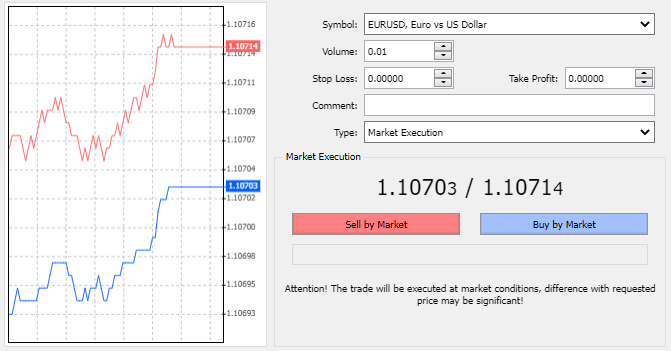As seen in the image above, EUR/USD has a bid price of 1.10703 and an asking price of 1.10714.

Given that 1 pip in a EUR/USD pair is in the 4th decimal place (0.0001), this would mean that this EUR/USD quotes has a 1-pip spread.

## What is the difference between a pip and a pipette?

A pip relates to movement in the fourth decimal place while a pipette is used to measure movement in the fifth decimal place. A pipette is a 'fractional pip' as it equals a tenth of a pip.

When looking at the difference between pip and pipettes in currency pairs involving the Japanese Yen, the pip relates to the second decimal point, and the pipette is the third decimal point.

## How many pips a day do forex traders make?

There is no set amount of pips you can make daily and will depend on your technical analysis, fundamental analysis, forex trading strategy, and ultimately, what way the market moves.

All traders want every day to be profitable but in the real world that doesn't exist as forex trading is very much a high-risk game. Stick to your trading plan, trial and innovate new strategies and practice proper risk management techniques.

## What are ticks and points?

A 'tick' is similar to a pip, but it may not measure every increment equally. For example, a tick on one instrument may be measured in increments of 0.0001 whereas another instrument may be measured in increments of 0.25.

A tick is simply the smallest increment a particular instrument can move in, and the terminology is usually used in securities or indices trading.

A point is another unit of measurement, used when there is a shift in the dollar amount. For example, if a share price went from \$25 to \$30, traders would say it has moved 5 points.

This term is also used in forex in place of 'pipette', to refer to the movement of the 5th decimal place.

## Pip and pipette

FAQ

### What is a pip in stocks?

With stock trading, pips are very rarely used as a term to define price movement since the shifts in stock prices move far more aggressively than they do in the foreign exchange market.

### What is a pip in crypto?

Pips are sometimes used in the crypto market to measure the movement in the price of a coin. Cryptocurrencies are traded at the dollar level, so a price movement of \$2,401 to \$2,402 would mean the cryptocurrency moved one pip.

In some cases, lower-value cryptocurrencies can utilise pips as units to measure cents or fractions of cents movement.Desmond Leong runs an award-winning research team (2019, 2020, 2021 Finalists for Best FX Research and Best Equity Research) advising the largest banks and brokers on where the markets are heading. He specialises in technical analysis with a focus on Fibonacci, chaos theory, correlations, market structure, and Elliott Wave.

Desmond is incredibly passionate about helping people become better traders - working closely with Axi to produce educational videos, quizzes, e-books, indicators, and market research to help traders take their game to the next level. His team is also behind the Axi VIP portal, dedicated to continuing to guide and educate traders.

Find him on:  LinkedIn | Instagram